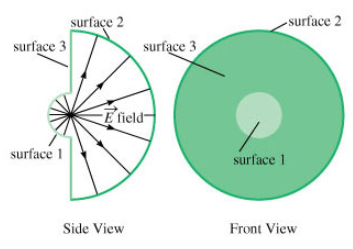# Problem: a) What is the electric flux Φ3 through the annular ring, surface 3? (Express your answer in terms of C, r1, r2, and any constants.)b) What is the electric flux Φ1 through surface 1? (Express Φ1 in terms of C, r1, r2, and any needed constants.)c) What is the electric flux Φ2 passing outward through surface 2? (Express Φ2 in terms of C, r1, r2, and any constants or other known quantities.)

🤓 Based on our data, we think this question is relevant for Professor Kokoouline's class at UCF.

###### FREE Expert Solution

Electric flux Φ = EAsinθ

Area of sphere As = 4πr2

So area of hemisphere Ah = 2πr2

(a) Surface 3 is parallel to the electric field: θ = 0 → sinθ = 0###### Problem Detailsa) What is the electric flux Φ3 through the annular ring, surface 3? (Express your answer in terms of C, r1, r2, and any constants.)

b) What is the electric flux Φ1 through surface 1? (Express Φ1 in terms of C, r1, r2, and any needed constants.)

c) What is the electric flux Φ2 passing outward through surface 2? (Express Φ2 in terms of C, r1, r2, and any constants or other known quantities.)